# Physics - Classical Mechanics - Periodic Motion Fundamentals

in STEMGeekslast year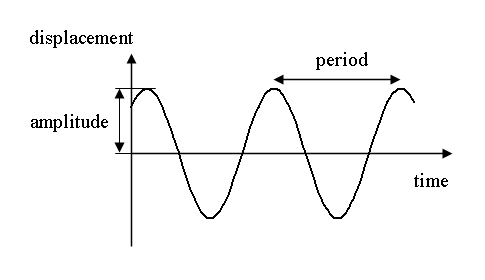[Image1]

## Introduction

Hey it's a me again @drifter1!

After almost a year has passed, it's time to continue with Physics, and more specifically the branch of "Classical Mechanics" (more than enough of a break!). We have finished the topic of Gravity when we covered Black Holes in the previous article on December 18th, 2020. The next chapter is Periodic Motion, which is also known as Oscillation. In this post we will start with a quick introduction to the Fundamentals of such motion!

So, without further ado, let's get straight into it!

## Fundamentals

A periodic motion is a motion that repeats itself over regular time intervals. One such complete repetion is known as a cycle.

### Period and Frequency

The duration of a complete cycle of oscillation is called a period and denoted by the letter T. The inverse, which is the number of cycles completed in a specific interval of time, is called frequency, and equal to the reciprocal of the period: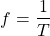The period is measured in seconds (s), whilst the frequency is measured in Hertz (Hz).

### Angular Frequency

In some motions its easier to use something known as angular frequency, denoted by the letter ω. This frequency refers to angular displacement per time (rad / s), and is given by: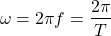### Hooke's Law and Return Force

The simplest oscillation can be expressed using spring deformation, and thus Hooke's Law. Let's say, that the center O in the coordinate system of such a mass on a spring system specifies the equilibrium position, and x specifies the deformation. Then a force, known as the return force, given by: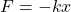will try to bring the system back to equilibrium.

### Acceleration and Velocity

From Newton's Second Law, the corresponding acceleration is: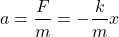The value of the acceleration is of course constantly changing! As the system comes close to the equilibrium position, the acceleration decreases, but the velocity increases. At equilibrium the velocity is maximum (acceleration is zero), whilst at maximum deformation the acceleration is maximum (velocity is zero).

### Amplitude

The maximum displacement (deformation) from the equilibrium position is known as the amplitude of oscillation, and commonly denoted by A. It's basically equal to the maximum value of |x|.

## Simple Harmonic Motion

A periodic motion which is described by Hooke's Law is known as a Simple Harmonic Motion.

In such a motion, the period of the mass on a spring is given by the equation: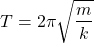The corresponding frequency is: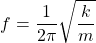Calculating the velocity, acceleration etc. for a specific amplitude, frequency etc. is more complicated. The equations that describes these quantities are solutions to differential equations, and will be covered later on!

## Example

Let's suppose a system with a spring of constant k = 500 N / m and a mass of m = 10 Kg.

• What's the corresponding return force for a deformation of x = 2 m?
• What's the acceleration applied to the mass on that position?
• What's the corresponding period of oscillation?

Substituting into Hooke's law, we easily calculate the return force: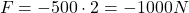The corresponding momentarily acceleration is equal to: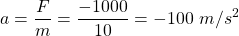Using the equation covered in the previous section, the period is: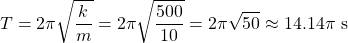which is independent of the amplitude and deformation. What changes is thus the maximum acceleration and velocity!

## Final words | Next up

And this is actually it for today's post!

Next time we will cover the energy in simple harmonic motion...

See ya!Keep on drifting!

Posted with STEMGeeks